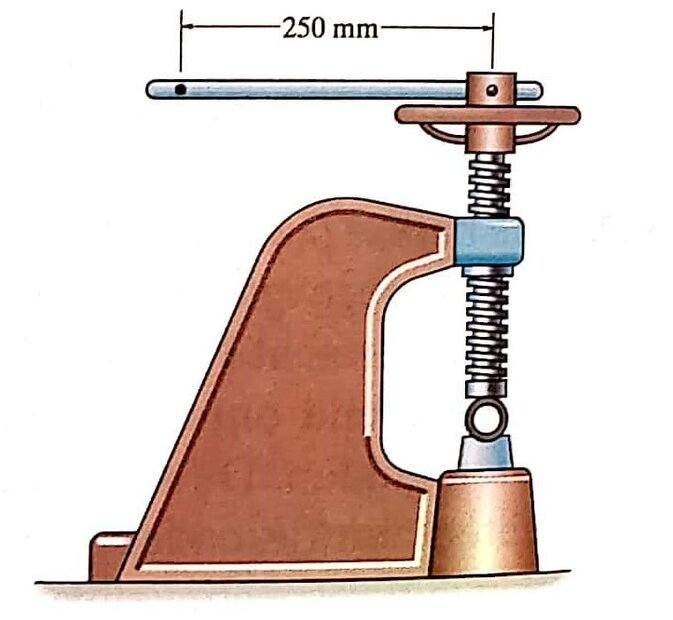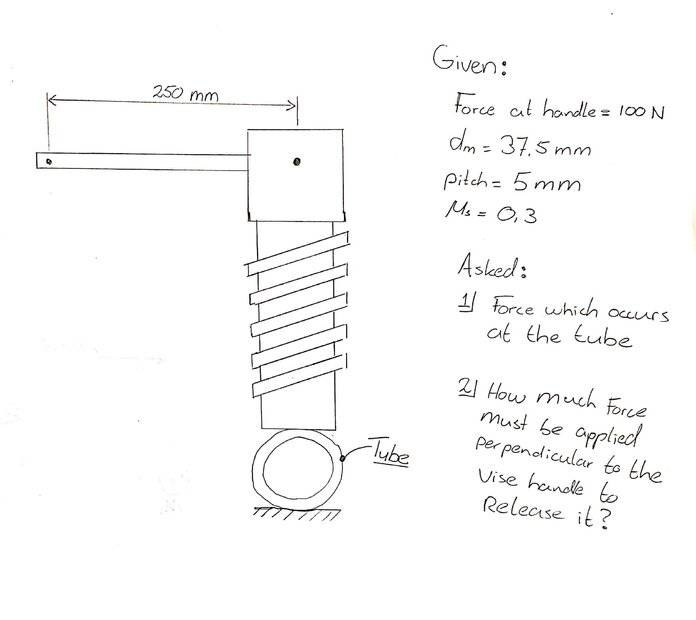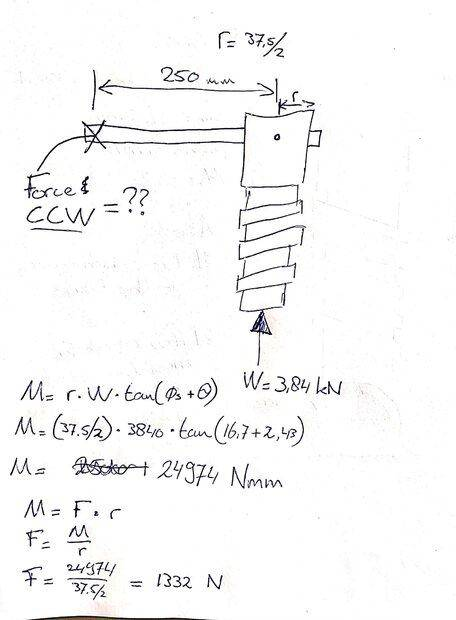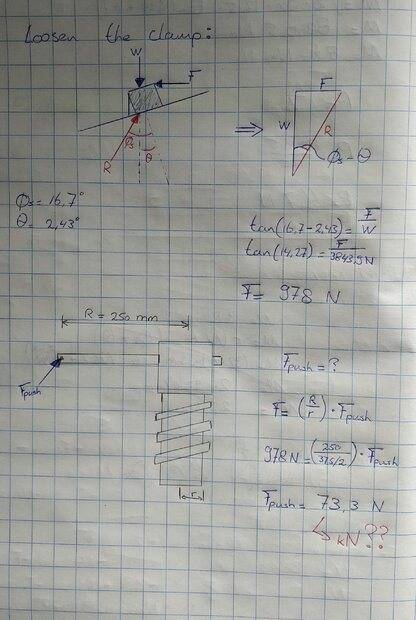# Torque applied on a vise screw (problem includes friction)

• Engineering
Homework Statement:
Friction applied on screws (power screw)
Relevant Equations:
M=r∙W∙tan⁡(∅s+θ)
θ=tan^(-1)⁡(l∕2πr)
∅s=tan^(-1)⁡〖(F∕N) = tan^(-1)⁡μs 〗
Hello all,

I need some help with a problem at statics, friction applied on threads. A threaded screw is given a ("horizontal") force of 100 N at the end of the handle. As the exercise states, they ask to calculate the force which occurs at the tube as well as the force needed to vise handle to release is.

With the formulas:
M=r∙W∙tan⁡(∅s+θ)
θ=tan^(-1)⁡(l∕2πr)
∅s=tan^(-1)⁡〖(F∕N) = tan^(-1)⁡μs 〗

First I calculated the Torque (which is M in the formula), M = 100 N * 250 = 25000 Nmm. The angles are found by using the mean diameter and friction coefficient of 0.3.
I founded the force W of 3.84 kN which is correct. But for the second question, the books gives a solution of 73.3 kN of force is needed to release the vise handle and I can't figure out how to solve this.

Hopefully someone can help.Lnewqban
Gold Member
In order to release the handle, a force will have to create a counter-clockwise torque of sufficient magnitude to overcome the static friction between the screw-nut threads that has been created by the axial force between vise and tube (disregarding friction between the tube and the end of the screw).

In order to release the handle, a force will have to create a counter-clockwise torque of sufficient magnitude to overcome the static friction between the screw-nut threads that has been created by the axial force between vise and tube (disregarding friction between the tube and the end of the screw).
Hi Lnewqban,

Thank you for the response :)

I tried to apply the notes you provided from the point of view I do understand. Unfortunately the outcome doesn't compare to the solution, see below:After a lot of attempts and this great video:
I have at least found amount of 73,3 N force needed to release the vise (see picture below). But to be honest, I can't place the steps taken to find the outcome. The Force F in the sketch is given in my book as: M/r (?). Besides, how did that formula F= (R/r)*Fpush arises? I found as outcome Fpush = 73,3 N instead of 73,3 kN??Hopefully someone could help here.

Thanks

Lnewqban
Gold Member
The input and output numbers of the problem do not match.
Without considering kinetic friction, the force on the tube should be 31416 N if applying the shown torque of 25 N-m.
Could you post a picture of the original text of the problem?

Last edited:
The text is in Dutch, makes that sense to you? - I have translated it below in English

"The machine clamp is used in order to hold the tube in position. At the end of the 250 mm long handle there will be a horizontal force of 100 N, determine the pressing force F that arises at the tube. The square threadings has an average diameter of 37.5mm and a pitch of 5 mm. How many force is perpendicular needed in order to release the handle? Suppose μs = 0.3"

Lnewqban
Gold Member
Thank you.
Does the book give you the correct answers for both forces?

Thank you.
Does the book give you the correct answers for both forces?
The book gives F = 3.84 kN (which I have correct), and P = 73.3 kN (I got 73.3 N here). I am doubting doing it on the correct way. I have both value's but can't verify the correct use of formulas...

Thank you.
Does the book give you the correct answers for both forces?
@Lnewqban, could you please explain how this problem should be solved in the correct way? I does not feel comfortable with the way of working I have done now and doesn't feel I do understand it yet...